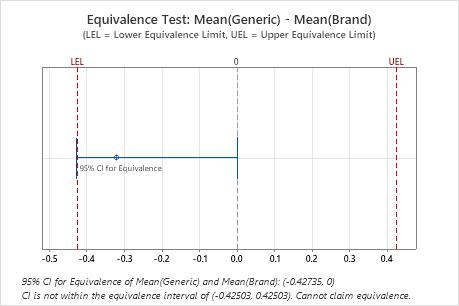# Interpret the key results for Equivalence Test for a 2x2 Crossover Design

Complete the following steps to interpret an equivalence test for a 2x2 crossover design. Key output includes the estimate of the effects, their p-values, the estimate of the difference (or ratio), the confidence interval, the equivalence plot, and other graphs.

## Step 1: Check for possible carryover or period effects

A significant carryover effect indicates that the treatment given in the first time period may affect the response to the other treatment given in the second time period. A significant period effect indicates that the period in which the treatment was given may affect the treatment response.
###### Important

If either the carryover effect or the period effect is statistically significant, you should not evaluate equivalence because your results may be biased by these effects.

Use the p-value for each effect to determine whether it is statistically significant. If the p-value for an effect is below the alpha level (typically, 0.05), then the effect is significant.

## Step 2: Determine whether the test mean and the reference mean are equivalent

Compare the confidence interval with the equivalence limits. If the confidence interval is completely within the equivalence limits, you can claim that the mean of the test population is equivalent to the mean of the reference population. If part of the confidence interval is outside the equivalence limits, you cannot claim equivalence.Key Results: 95% CI, Equivalence interval In these results, the 95% confidence interval is not completely within the equivalence interval defined by the lower equivalence limit (LEL) and the upper equivalence limit (UEL). The lower bound of the confidence interval extends beyond the lower equivalence limit. Therefore, you cannot conclude that the test mean is equivalent to the reference mean.
By using this site you agree to the use of cookies for analytics and personalized content.  Read our policy